# Half Square Triangles

Tags: half square triangle, how-to, patchwork, quilting lesson

Learn how to make half square triangle blocks as well as learning where to use half square triangles.

## What are Half Square Triangles?

A Half Square Triangle is a square that is cut through the diagonal to give two triangles. Two half square triangles make a square.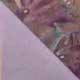half square triangle

Cut two squares of fabric the required size. For this lesson, we will use 3" squares.

On the lighter fabric, draw a line from upper corner to lower corner through the center point of the square.

Place both squares, right sides together, and stitch ¼" on both sides of the drawn line. Cut along line.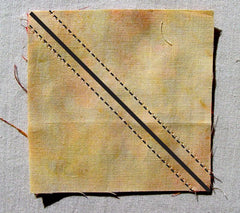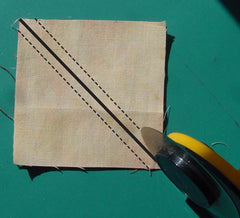Press seam as it is. This sets the stitches and keeps the block in shape.

Place block, withdarkest square on top, on ironing board. Lift top triangle and using the iron, press it over seam.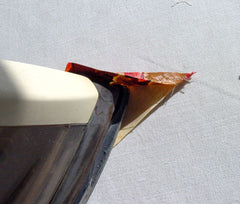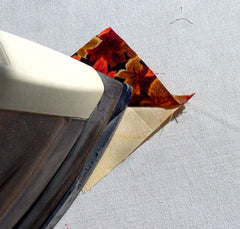Using a square ruler, match diagonal line with seam line and trim block to a 2½" square.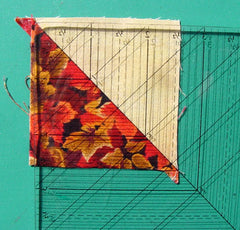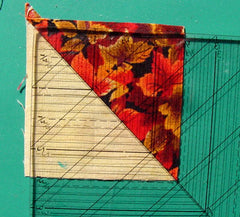One set of squares makes two half square triangle blocks.

## Why should we not cut the squares before we stitch them?

Stitching before cutting is a much more accurate way of making half square triangles. Since we are stitching on the bias of the fabric, the seam can stretch easily. By stitching through the square, there is less chance of the seam stretching out of shape, making a much more
accurate block.

## How do you determine the cutting size?

Add 1" to the finished size of the block. This example shows an 8" block. The dotted lines indicate 2" grid. Half square triangles (outlined in red) for this block are 2" therefore cut each square 3".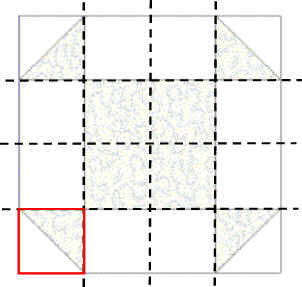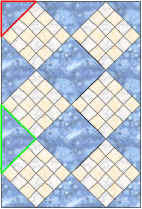## Where else do we use half square triangle?

Half square triangles can also be used for corner setting triangles of a quilt made where blocks are on-point (outlined in the red triangle). Here
we just use single triangles so they are cut before stitching.

### Do we also use single half square triangles for the setting triangles on the side of a quilt made with blocks on-point?

No. We use quarter square triangles for this (quarter square triangle is outlined in green).

### Why do we use half square triangles for the corner setting triangles and quarter square triangles for the sides of an on-point quilt?

This is so when we add borders or binding, we are stitching along the grain of the fabric rather than the bias.

### Why is it necessary to apply borders on the straight of grain instead of the bias?

This reduces the likelihood of the quilt top stretching out of shape while stitching. If the quilt top stretches, it can create wavy borders on your quilt.

Check out this pattern that uses half square triangles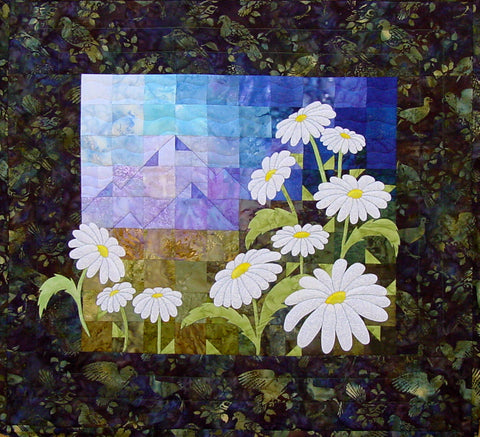We have many online classes, you might like to check them out here: online classes >>

© Copyright 2003- Arbee Designs. All Rights Reserved. This content is copyrighted by the respective teachers or authors of articles or lessons presented on this page. Unless otherwise indicated, all other content is the property of Arbee Designs. Copies of the material for others may not be made without the permission of Arbee Designs.